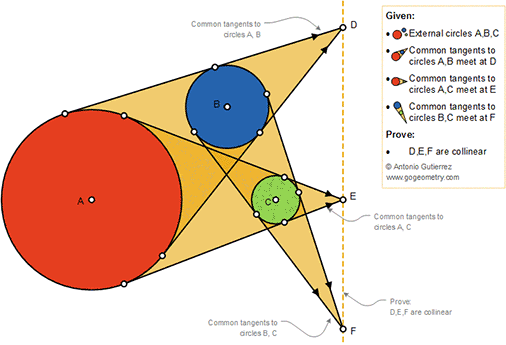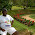## Sunday, October 7, 2018

### Geometry Problem 1393: Monge's Circle Theorem, Three Circles and Three Pair of Common Tangents, Collinearity

Geometry Problem. Post your solution in the comment box below.
Level: Mathematics Education, High School, Honors Geometry, College.

Details: Click on the figure below.#### 2 comments:

1.AD / BD = rA / rB
CE / AE = rC / rA
BF / CF = rB / rC

Multiplying the 3 equations RHS = 1
Hence applying Menelaus the result follows

Sumith Peiris
Moratuwa
Sri Lanka

2.Let radius of circles centered A, B and C are r1, r2 and r3
Connect DAB, ECA and FCB
We have DB/DA= r2/r1
EA/EC= r1/r3
FC/FB= r3/r2
Multiply above expressions side by side we have DB/DA x EA/EC xFC/FA= 1
So D, E, F are collinear per Menelaus theorem